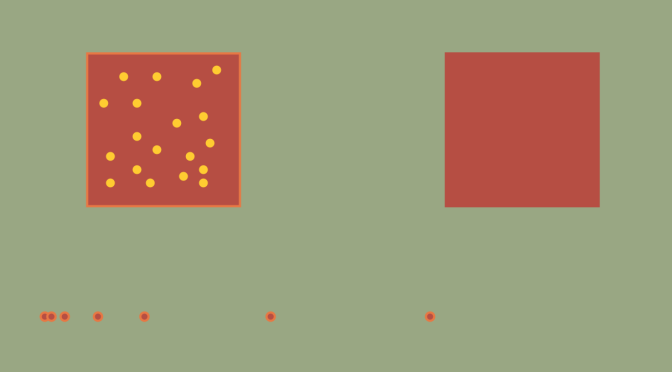# Playing with interior and closure

Let’s play with the closure and the interior of sets.

To start the play, we consider a topological space $$E$$ and denote for any subspace $$A \subset E$$: $$\overline{A}$$ the closure of $$A$$ and $$\overset{\circ}{A}$$ the interior of $$A$$.

### Warm up with the closure operator

For $$A,B$$ subsets of $$E$$, the following results hold: $$\overline{\overline{A}}=\overline{A}$$, $$A \subset B \Rightarrow \overline{A} \subset \overline{B}$$, $$\overline{A \cup B} = \overline{A} \cup \overline{B}$$ and $$\overline{A \cap B} \subset \overline{A} \cap \overline{B}$$.

Let’s prove it.
$$\overline{A}$$ being closed, it is equal to its closure and $$\overline{\overline{A}}=\overline{A}$$.

Suppose that $$A \subset B$$. As $$B \subset \overline{B}$$, we have $$A \subset \overline{B}$$. Also, $$\overline{B}$$ is closed so it contains $$\overline{A}$$, which proves $$\overline{A} \subset \overline{B}$$.

Let’s consider $$A,B \in E$$ two subsets. As $$A \subset A \cup B$$, we have $$\overline{A} \subset \overline{A \cup B}$$ and similarly $$\overline{B} \subset \overline{A \cup B}$$. Hence $$\overline{A} \cup \overline{B} \subset \overline{A \cup B}$$. Conversely, $$A \cup B \subset \overline{A} \cup \overline{B}$$ and $$\overline{A} \cup \overline{B}$$ is closed. So $$\overline{A \cup B} \subset \overline{A} \cup \overline{B}$$ and finally $$\overline{A \cup B} = \overline{A} \cup \overline{B}$$.

Regarding the inclusion $$\overline{A \cap B} \subset \overline{A} \cap \overline{B}$$, we notice that $$A \cap B \subset \overline{A} \cap \overline{B}$$ and that $$\overline{A} \cap \overline{B}$$ is closed to get the conclusion.

However, the implication $$\overline{A} \subset \overline{B} \Rightarrow A \subset B$$ doesn’t hold. For a counterexample, consider the space $$E=\mathbb R$$ equipped with the topology induced by the absolute value distance and take $$A=[0,1)$$, $$B=(0,1]$$. We have $$\overline{A}=\overline{B}=[0,1]$$.

The equality $$\overline{A} \cap \overline{B} = \overline{A \cap B}$$ doesn’t hold as well. For the proof, just consider $$A=[0,1)$$ and $$B=(1,2]$$.

### Can $$\overline{A \cap B}$$, $$\overline{A} \cap B$$, $$A \cap \overline{B}$$ and $$\overline{A} \cap \overline{B}$$ be all different?

The answer is positive. We take again $$E=\mathbb R$$ with $$A=[0,1) \cup [2,3)$$ and $$B = [1,2)$$.
Then $$\overline{A}=[0,1] \cup [2,3]$$ and $$\overline{B}=[1,2]$$, which gives:
$$\overline{A \cap B} = \emptyset$$, $$\overline{A} \cap B = \{1\}$$, $$A \cap \overline{B}=\{2\}$$ and $$\overline{A} \cap \overline{B}=\{1,2\}$$

### Can you find $$A \subset \mathbb R^2$$ such that $$A, \overline{A}, \overset{\circ}{A}, \overset{\circ}{\overline{A}}, \overline{\overset{\circ}{A}}$$ are all different?

Let’s build $$A$$ by steps.

First, $$A$$ cannot be closed nor open. For this, we pick-up the set consisting of the elements of a converging sequence whose values are distinct from the limit as: $A_1=\{u_n=(\frac{1}{n},2) \ : \ n \in \mathbb N\}$ We also need to have $$\overset{\circ}{A} \neq \overset{\circ}{\overline{A}}$$. This can be achieved by taking a dense subset of a closed subset of $$\mathbb R^2$$, like $A_2=[0,1] \cap \mathbb Q^2$ We have $$A_2= \emptyset$$, $$\overline{A_2}=[0,1]^2$$ and $$\overset{\circ}{\overline{A_2}}=(0,1)^2$$.

Finally, $$\overset{\circ}{A}$$ and $$\overline{\overset{\circ}{A}}$$ have to be distinct, which can be achieved by taking the open square $A_3=(3,4)^2$ for which $A_3=(3,4)^2, \overset{\circ}{A_3}=A_3 \text{ and } \overline{A_3}=[3,4]^3$ Based on those subsets, we take $$A= A_1 \cup A_2 \cup A_3$$. Then: \begin{align*} A &= A_1 \cup A_2 \cup A_3 \\
\overline{A} &= A_1 \cup \{(0,2)\} \cup [0,1]^2 \cup [3,4]^2\\
\overset{\circ}{A} &= (3,4)^2 \\
\overset{\circ}{\overline{A}} &= (0,1)^2 \cup (3,4)^2 \\
\overline{\overset{\circ}{A}} &= [3,4]^2
\end{align*}